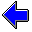Java Security Settings:
This web page employs Java, which requires specific security settings for correct operation.
If the applets on this page do not run correctly, consult the Virtual Chemistry Experiments FAQ
or the Physlet Physics web site for establishing the correct security settings.

## Molecular Orbital Diagrams

### Concepts

In Molecular Orbital Theory, the orbitals in a molecule arise from the overlap of atomic orbitals that satisfy two criteria:

• The orbitals have compatible symmetry
• The orbitals are similar in energy

The interactions of the atomic orbitals to form molecular orbitals is represented by an energy diagram called a molecular orbital diagram. The diagram displays the orbitals arranged vertically from lowest to highest energy. The atomic orbitals for the various atoms are listed in columns at the left and right sides of the diagram. The molecular orbitals are listed in a column down the center of the diagram.

The atomic orbitals are given the usual s, px, etc. designation. The molecular orbitals, which are often identified by their symmetry properties, may also be identified by their bonding properties: σ, σ*, π, π*, or n.

In this exercise you will be presented with the molecular orbital diagram for a molecule. Each atomic orbital is identified by its proper designation, but the molecular orbitals are given a generic, nondescriptive designation. Clicking upon a particular orbital on the diagram displays the isosurface for that orbital in the virtual reality window at the right. For linear molecules bonding is defined to occur along the z axis. In general, the coordinate system is defined so that the z axis is the highest axis of symmetry. The positions of the nuclei are shown by small colored dots.

Carefully examine each of the molecular orbitals and answer the following questions.

1. For each molecular orbital, indicate whether the orbital is σ, σ*, π, π*, or n.
2. For each molecular orbital, identify the atomic orbital or orbitals that "mixed" to form that molecular orbital.
3. For each molecular orbital, identify the atom on which the orbital is primarily located. If the orbital is equally distributed around several atoms, identify each atom.
4. Write the electron configuration for the molecule. When doing this, replace the MO 1, MO 2, etc. terms with the appropriate orbital designations: σ, σ*, π, π*, or n. If there are multiple σ orbitals, for example, use the designations σ1, σ2, etc..
5. Use the molecular orbital diagram to determine the bond order for each chemical bond. Consider all orbitals that serve to "connect" two atoms. Subtract the total number of electrons in anti-bonding orbitals from the total number of electrons in bonding orbitals. Divide the result by two to obtain the bond order. (Does it make sense that electrons in non-bonding orbitals would be ignored in determining the bond order?) A bond order of 1.0 corresponds with a single bond in a Lewis structure. A bond order of 2.0 corresponds with a double bond in a Lewis structure.
6. Compare the molecular orbital representation of the bonding with the Lewis structure. Are the two models consistent in their description of the bonding?

### Molecular Orbital Diagram of the Dihydrogen Molecule

The molecular orbital diagram for the H2 molecule is shown at the lower left. Click on each of the orbitals to view the corresponding orbital isosurface in the virtual reality box at the lower right.

The dotted lines are used to connect molecular orbitals with the atomic orbitals that "mixed" to form the molecular orbital.

Whenever two atomic orbitals mix, the result is two molecular orbitals of which one has bonding character and the other has anti-bonding character. The overlap of the two hydrogen 1s orbitals produces two molecular orbitals: MO 1 and MO 2. The lower energy orbital is the bonding orbital; the higher energy orbital is the anti-bonding orbital.

Examination of the isosurface for MO 1 shows that electron density is concentrated in the region immediately between the two nuclei. Thus MO 1 is a sigma bonding orbital (σ).

Examination of the isosurface for MO 2 shows that electron density is concentrated away from the region immediately between the two nuclei. In this case the electron density is pushed to the exterior of the molecule and there is a nodal surface separating the two nuclei. Thus MO 2 is a sigma anti-bonding orbital (σ*). The designation is sigma, because the electron density still is centered on the line containing the two nuclei.

Owing to the symmetry of the molecule, the electrons in both the σ and σ* orbitals are uniformly distributed between the two atoms.

The electron configuration for H2 is (σ)2. The bond order is thus 1.0.View Along     Bonding is along the z axis.

Drag with the left mouse button to rotate, the center button to zoom, and the right button to move the object.

### MoleculesMolecular Orbital Theory Home PageVirtual Chemistry Experiments Home Page

MO.html version 2.1